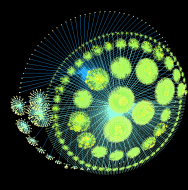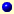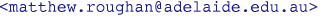# Complex-Network Modelling and Inference

 HomeNewsLecture NotesHandoutsAssignmentsTutorialsMatlab (protected) filesDataOther stuff
Assignments
 Assignment 1 (Graph basics) [PDF] Assignment 2 (Node degree and Eulerian paths) [PDF] Assignment 3 (Graph tricks and tools) [PDF] Assignment 4 (Random Graphs) [PDF] Assignment 5 (Graph Operators) [PDF] Assignment 6 (Modelling) [PDF] Assignment 7 (Path Algebras) [PDF] Assignment 8 (Competition) [PDF] Assignment 9 (Data and Modelling) [PDF]

Matthew Roughan